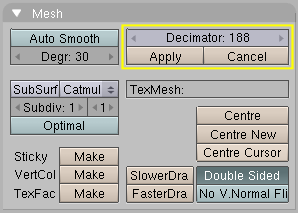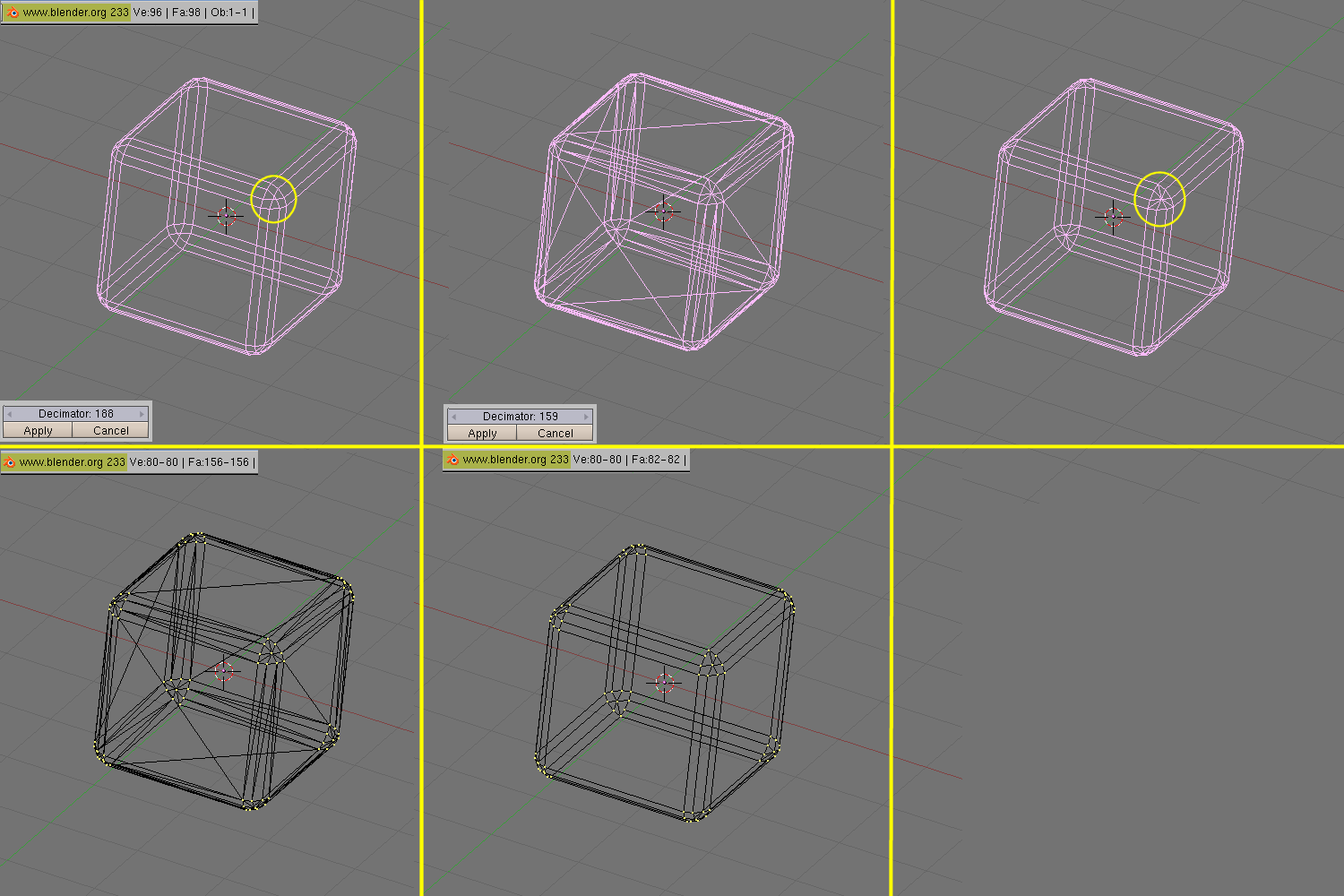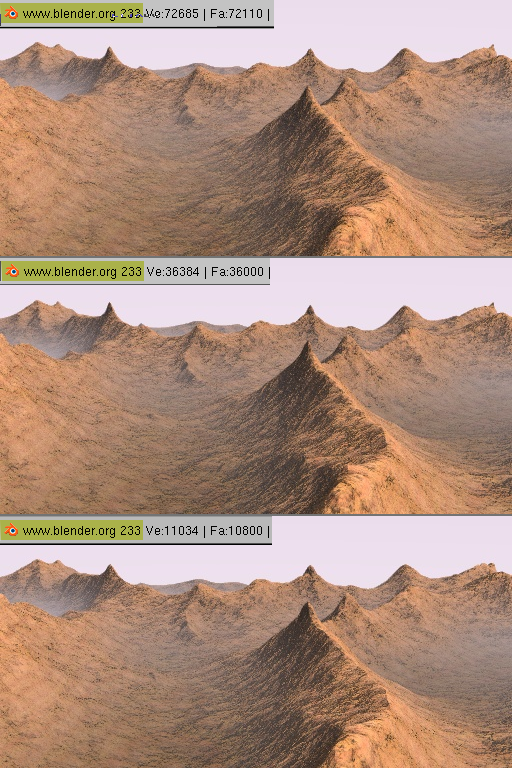# Decimator Tool

Relevant to Blender v2.33

The Decimator tool is an often overlooked feature which allows you to reduce the vertex/face count of a mesh with minimal shape changes.

This is not applicable to meshes which have been created by modelling carefully and economically, where all vertices and faces are necessary to correctly define the shape, but if the mesh is the result of complex modelling, with proportional editing, successive refinements, possibly some conversions from SubSurfed to non-SubSurfed meshes, you might very well end up with meshes where lots of vertices are not really necessary.

A simple example is a plane, and a 4x4 undeformed Grid object. Both render exactly the same, but the plane has 1 face and 4 vertices, while the grid has 9 faces and 16 vertices, hence lots of unneeded vertices and faces.

The Decimator Tool (Figure 30) allows you to eliminate these unneeded faces. Its NumButton reports the number of faces of the selected mesh in ObjectMode. The decimator tool only handles triangles, so each quadrilateral face is implicitly split into two triangles for decimation.Figure 30. Decimator buttons.

Let's consider the example we used in the Bevel section. As you might notice there is a tiny triangular face on each cube vertex which might very well be unnecessary (Figure 31, top left). The header says the cube has 98 faces and 96 vertices. The Decimator button says the cube has 188 triangular face, namely 90 quads (which are 180 tris) and 8 tris.Figure 31. Decimator at work.

By changing the number in the decimator NumBut, by either clicking or typing it in, the mesh immediately changes to triangles only. As the number gets lower, faces disappear one after the other. Blender causes coplanar faces and vertices on aligned edges to disappear first. This tends to keep the shape of the mesh. As more and more faces are asked to be removed faces less and less coplanar and vertices less and less colinear are merged, hence sensible shape change might occur (Figure 31, top center).

In this particular case, if we just want the central tri face of each cube vertex to disappear we expect the final mesh to be 2x6=12 faces for each cube face, 2x3x12=72 faces for each bevelled edge, and 9x8=72 faces for each bevelled vert, totalling 156 faces. It is very uncommon to know beforehand how many faces the final mesh can have, usually you must look carefully at the mesh in a 3D window to check that the shape is still good.

The two buttons below the Decimator finalize or cancel the decimation. Once it is finalized triangles are not shown any more (Figure 31, top right) but the mesh is nevertheless made only of triangles (Figure 31, bottom left). You can revert to quads if you so wish, by selecting all vertices and hitting ALT-J (Figure 31, bottom center). This way we reduce the vertex count to 80 and face count to 82 without any noticeable shape loss. It might look a small gain, but if this cube is going to be dupliverted on a particle system with 1000 particles it might be worth it.Figure 32. Decimated landscape, top: original; middle: lightly decimated; bottom: heavily decimated.

Figure 32 shows a landscape generated via a careful application of the Noise technique described earlier, on a quite vast grid. On top, the result for the original mesh and below, two different levels of decimation. To the eye the difference is indeed almost unnoticeable, but as the vertex count goes down there is a huge gain.